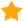2 doctors weighed in:

# I would like help to calculate peak blood flow rate in aorta when given radius and peak blood velocity?

2 doctors weighed in
Dr. Bennett Werner
Internal Medicine - Cardiology
1 doctor agrees

## In brief: Bernoulli Equation

For peak pressure, you only need peak velocity.
Peak pressure is 4 x the square of the peak velocity. If the peak velocity is 4 m/s, the peak pressure is 64 mmHg. The peak velocity IS the peak flow rate. For example, if peak velocity is 5 l/min, then the peak flow rate is 5 l/min. Is that what you meant?

## In brief: Bernoulli Equation

For peak pressure, you only need peak velocity.
Peak pressure is 4 x the square of the peak velocity. If the peak velocity is 4 m/s, the peak pressure is 64 mmHg. The peak velocity IS the peak flow rate. For example, if peak velocity is 5 l/min, then the peak flow rate is 5 l/min. Is that what you meant?
Get help from a real doctor now
Dr. Tonga Nfor
Board Certified, Internal Medicine - Cardiology
14 years in practice
508K people helped111,000 doctors available